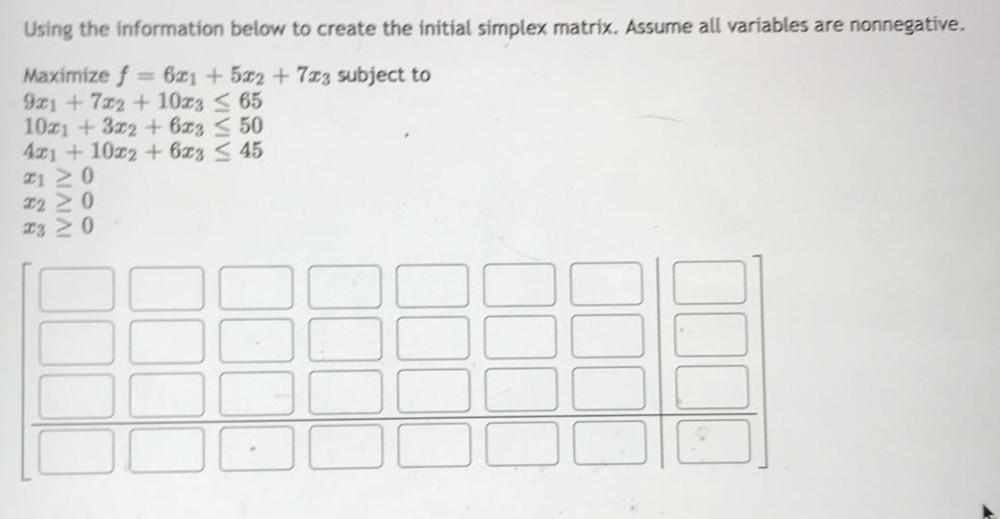Question:

# Using the information below to create the initial simplex matrix. Assume all variables are nonnegative. Maximize f = 6x₁ +5x₂ + 7x₃ subject to 9x₁ + 7x₂ + 10x₃ ≤ 65 10x₁ + 3x₂ + 6x₃ ≤ 50 4x₁ + 10x₂ +Using the information below to create the initial simplex matrix. Assume all variables are nonnegative. Maximize f = 6x₁ +5x₂ + 7x₃ subject to 9x₁ + 7x₂ + 10x₃ ≤ 65 10x₁ + 3x₂ + 6x₃ ≤ 50 4x₁ + 10x₂ + 6x₃ ≤ 45 x₁ ≥0 x₂ ≥ 0 x₃ ≥ 0